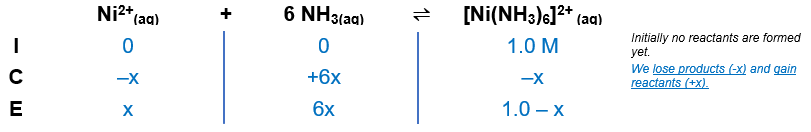# Problem: Calculate the equilibrium concentration of Ni2+ in a 1.0-M solution [Ni(NH3)6](NO3)2.

###### FREE Expert Solution

[Ni(NH3)6](NO3)2→ nitrates are soluble

[Ni(NH3)6](NO3)2(aq)[Ni(NH3)6]2+(aq) + 2 NO3-(aq)

Ni2+(aq) + 6 NH3(aq) [Ni(NH3)6]2+(aq)

Kf [Ni(NH3)6]2+ = 5.5×108

Construct an ICE chart:${\mathbf{K}}_{\mathbf{f}}\mathbf{=}\frac{{\left[\mathrm{Ni}{\left({\mathrm{NH}}_{3}\right)}_{6}\right]}^{\mathbf{2}\mathbf{+}}}{\left[{\mathrm{Ni}}^{2+}\right]{\left[{\mathrm{NH}}_{3}\right]}^{\mathbf{6}}}\phantom{\rule{0ex}{0ex}}\mathbf{5}\mathbf{.}\mathbf{5}\mathbf{×}{\mathbf{10}}^{\mathbf{8}}\mathbf{=}\frac{\mathbf{1}\mathbf{.}\mathbf{0}\mathbf{-}\mathbf{x}}{\left(x\right){\left(6x\right)}^{\mathbf{6}}}$###### Problem Details

Calculate the equilibrium concentration of Ni2+ in a 1.0-M solution [Ni(NH3)6](NO3)2.